1) The originally compressed gas from a carbon dioxide fire extinguisher emerges so rapidly that no immediate energy transfer occurs by heat between the gas and the atmosphere. Use this information to explain why the escaping gas is very cold.

2) A thermodynamic process occurs in which the entropy of a system changes by -8.0 J/K. According to thelaw of thermodynamics, what can you conclude about the entropy change of the environment?

3) One mole of an ideal gas initially at a temperature of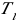=0° C undergoes an expansion at a constant pressure of one atmosphere to four times its original volume.

A. Calculate the new temperature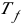of the gas.

B. Calculate the work done on the gas during the expansion.

4) In one cycle, a heat engine absorbs 500 J from the high temperature reservoir and expels 300 J to a low temperature reservoir. If the efficiency of this engine is 60% of the efficiency of a Carnot engine, what is the ratio of the low temperature to the high temperature in the Carnot engine?

5) An electrical power plant has an overall efficiency of 15%. The plant is to deliver 150 MW of electrical power to a city and its turbines use coal as fuel. The burning coal produces steam at 190°C, which drives the turbines. This steam is then condensed into water at 25°C by passing through coils in contact with river water.

A. How many metric tons of coal does the plant consume each day (1 metric ton = 1 X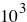kg)?

B. What is the total cost of the fuel per year if the delivered price is \$8 per metric ton?

C. If the river water is delivered at 20°C, at what minimum rate must it flow over the cooling coils in order that its temperature not exceed 25°C? (NOTE The heat of combustion of coal is 7.8 Xcal/g)

6) A gas is compressed at a constant pressure of 0.800 atm from 9.00 L to 2.00 L. In the process, 400 J of energy leaves the gas by heat.

A. What is the work done ON the gas?

B. What is the change in its internal energy?

7) A 100 kg steel support rod in a building has a length of 2.0 m at a temperature of 20°C. The rod supports a hanging load of 6000 kg. Find

A. the work done ON the rod as the temperature increases to 40°C.

B. The energy Q added to the rod (assume the specific heat of steel is the same as that for iron)

C. The change in internal energy of the rod

8) Find the Celsius temperature change of a river due to the exhausted energy from a nuclear power plant. Assume that the input power to the boiler in the plant is 25 X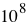W, the efficiency of the use of this power is 30%, and the river flow rate is 9.0 X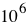kg/min.

9) One mole of neon gas is heated from 300 K to 420 K at constant pressure. Calculate

A. the energy Q transferred to the gas

B. the change in the internal energy of the gas

C. the work done on the gas

(NOTE neon has a molar specific heat of c = 20.29 J/mol-K for a constant-pressure process)

10) A 5.0 kg block of aluminum is heated from 20°C to 90°C at atmospheric pressure. Find

A. the work done by the aluminum

B. the amount of energy transferred to it by heat

C. the increase in its internal energy

(source)